﻿ Magnetic force and de Broglie waves.

# Magnetic force and de Broglie waves.

## Magnetic force is caused by de Broglie wave, NOT by unreal virtual photons.

(Fig.1)  "Virtual" photons in QED is "unreal", de Broglie wave is realistic.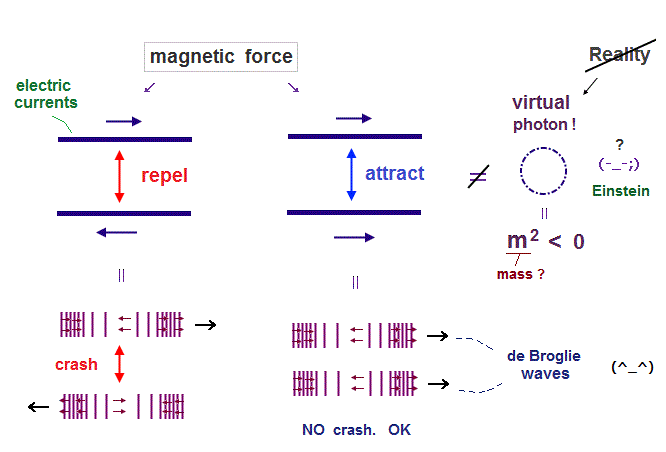The current quantum mechanics argues that Coulomb force is caused by ( unreal ) virtual photons.  See this, this.  And they say the magnetic force is also due to virtual photons. See this, this.

In Feynman diagram, the square of mass of virtual photon always becomes negative ( m2 < 0 ), which means this virtual photon is faster-than-light tachyon. So unreal.  See this, this.

The important point is that the progress in our science stops in these virtual particles, because they are "virtual" (= NOT real ).  Physicists avoid clear pictures and property of virtual particles, forever. See this, this.

It is known that a moving electron (= electric current ) generates de Broglie waves.
So it is quite natural that we think this magnetic force is caused by de Broglie wave interference rather than unrealistic virtual photons.

## One de Broglie wavelength consists of low and high pressure areas.

(Fig.2)  One de Broglie wavelength of electron.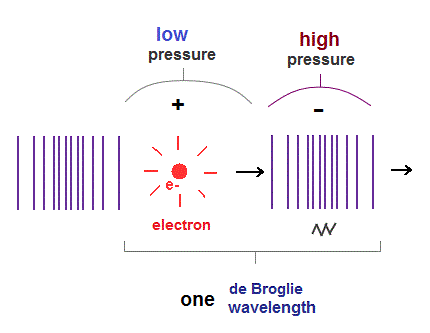When an electron moves, electric fields (= E ) around the electron also moves with it.
So this spreading electric field causes de Broglie waves, which can be confirmed by Davisson-Germer experiment.

We can naturally think this de Broglie wave is a kind of longitudinal waves through some medium.
If so, double slit interference can be explained by this "real" de Broglie waves, NOT by "fantasy" many-worlds.

Longitudinal wave consists of "low" (= sparse ) and "high" (= dense ) pressure areas.
It is thought that an electron pushes the field around it toward "dense" area, and is moving with "low" pressure area.

## Oscillating directions inside de Broglie waves.

(Fig.3)  "Dense" area oscillates in propagation direction, "sparse" area is the opposite.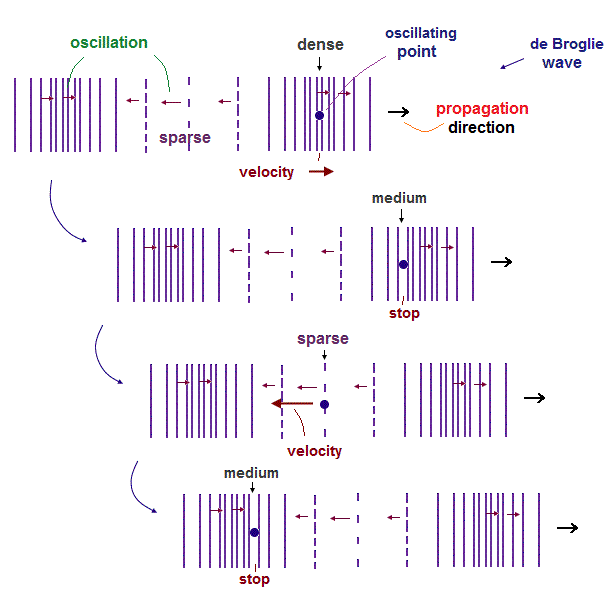As shown in this and this, the field included in "dense" area oscillates in the propagation direction, and the field in "sparse" area oscillates in the opposite direction.

For the total amount of currents in both directions to be the same, the velocity in the "sparce" field is higher than that of "dense" area, because there are less obstacles in "sparse" area.

Each "field particle" stops on both ends of its oscillation, which have "medium" density.

## "Opposite" de Broglie waves crash and repel each other.

(Fig.4)  The same phase areas have the "opposite" oscillations in the "opposite" waves.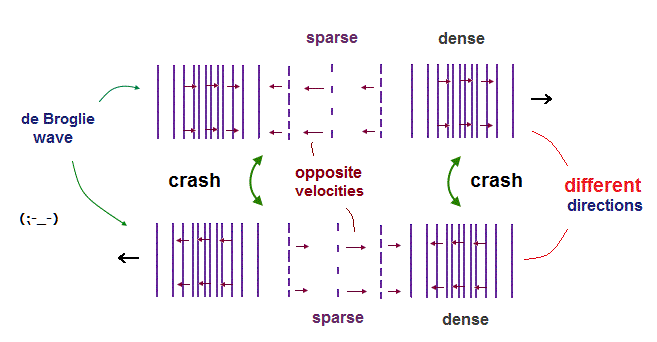So, when two de Broglie waves in the opposite directions approach each other, the oscillating velocities of the same phase areas ( sparse-sparse, dense-dense ) become the opposite.

So both fields crash into and "repel" each other, when two de Broglie waves propagate in the opposite directions.

## Magnetic force between electric currents can be explained by de Broglie waves.

(Fig.5)  Magnetic (= Lorentz ) force between two wires = de Broglie waves.As shown on this site and this, according to Lorentz magnetic force, two wires carrying electric currents in the same direction attract each other.  And the opposite currents repel each other.

The electric current is the flow of ( moving ) charged particles (= electron ).
Moving electrons generate their de Broglie waves, so it is clear this de Broglie wave has something to do with magnetic force !

## Opposite electron's de Broglie waves (= currents ) repel each other.

(Fig.6)  The opposite de Broglie waves (= currents ) have the "opposite" oscillating velocities.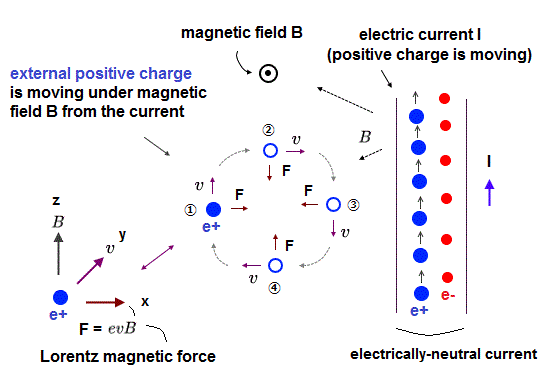As I said, electron's de Broglie waves propagating in the opposite directions contain the opposite oscillating fields in the same-phase areas, so these opposite waves repel each other.

The electric currents are moving electrons (= de Broglie waves ).
So the repulsive de Broglie waves express the electric currents repelling each other by magnetic forces.

## de Broglie waves (= currents ) in the "same" direction "attract" each other.

(Fig.7)  de Broglie waves in the same direction contain the same oscillation.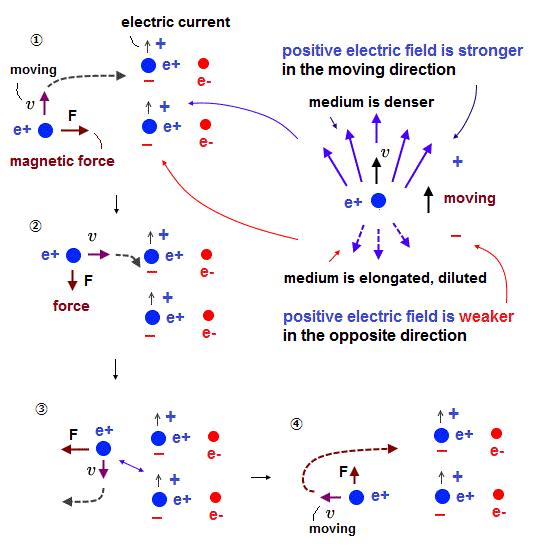When two de Broglie waves propagate in the same direction, their oscillations are the same and harmonize with each other (= constructive interference ).

As a result, two de Broglie waves ( electric currents ) in the same direction attract each other, which is thought to be Lorentz "magnetic" force.  It is similar to Magnus effect.

## "Moving" electric fields (= de Broglie waves ) and magnetic force.

(Fig.8)  Moving electric fields with different strength cause different magnetic force.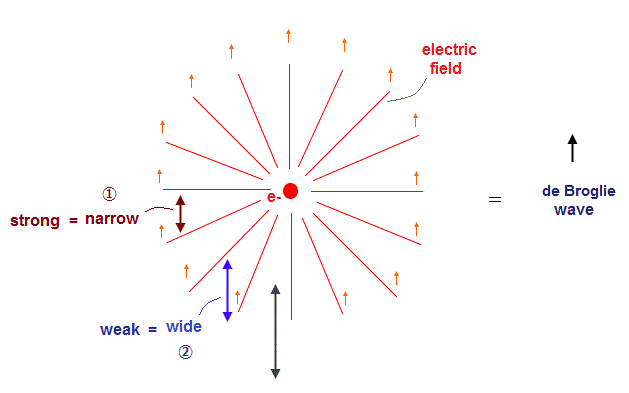Of course, the electric field lines radiate outwards ( or inwards ) from electric charges.
If we suppose the de Broglie waves are caused by moving ( or propagting ) electric fields, we have to consider the difference in the distribution of electric field lines.

In Fig.8, an electron and its electric field are moving upward.
In the left area (= "1" ), the spaces of electric field is narrow in the propagation direction, so the fields can be more compressed.

So the generated de Broglie waves become stronger (= more compressed ) in the narrow electric fields than the wide electroc fields (= "2" ).

## Biot-Savrt law can be explained by de Broglie waves.

(Fig.9)  Biot-Savart law -- magnetic and electric fields.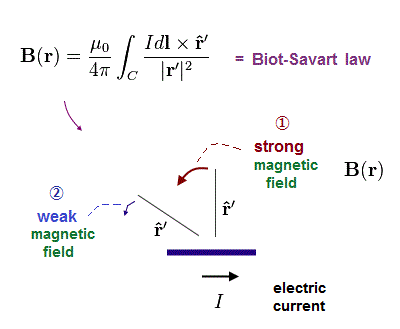The relation between electric fields and de Broglie waves can naturally explain Biot-Savart law.
In Biot-Savart law, the magnetic fields become stronger at points (= "1" in Fig.9 ) which are perpendicular to the electric currents.

This relation between magnetic force strength and currents is just equal to that between the electric field's spaces (= narrow or wide ) and de Broglie waves in Fig.8.

## How longitudinal de Broglie wave is generated in the "field" ?

(Fig.10)  Moving charges "push" the rest field.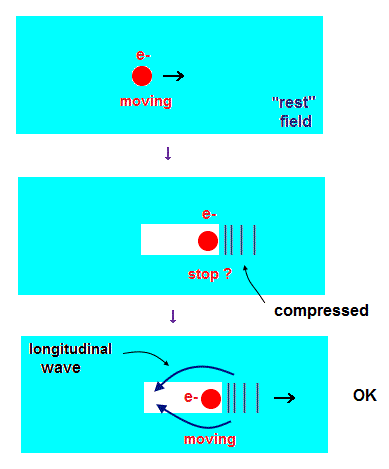Considering fatal paradoxes of special relativity, it is natural we think some aether-like matter moving with the earth can explain Michelson-Morley experiments.

In this rest field ( with respect to the earth ), when a charge ( and its electric field ) starts to move, it pushes and compresses the fields.

This compressed field causes longitudinal waves, and oscillates back, passing charge.
As a result, this charge can move with "sparse" area of this longitudinal waves smoothly.

## Conservation of total energy assures each particle's velocity.

(Fig.11)  Two particles can keep their velocities due to energy conservation.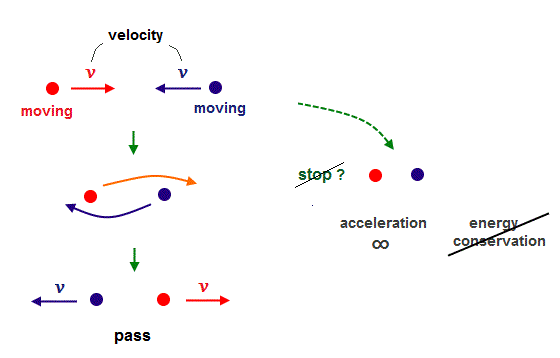Even when two particles with the opposite velocities (= ±v ) crash into each other, they never stop ( if potential energies or field densities do not change ).

Because if they suddenly stop after collision, it violates energy conservation and needs "infinite" acceleration.

So if the total energy is conserved ( like elastic collision ), two particles can move with the same speeds as before collision.

## As "mass" and "velocity" are greater, de Broglie wavelength becomes shorter.

(Fig.12)  de Broglie wavelength becomes shorter, as momentum is bigger.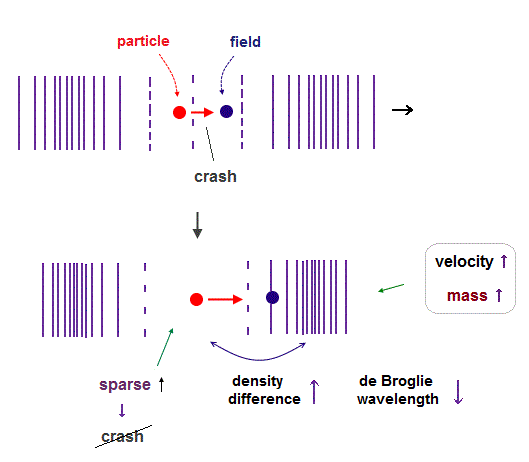Even if the velocities of charged particles are the same, its momentums becomes bigger as its mass is greater. Because bigger mass tends to push and compress more fields.

On this page, we can naturally think the large difference between electron and proton masses are due to their "density" difference (= heavy proton has much denser fields ).

So Coulomb attractive force is caused by "dense" positive proton is attracted toward "sparse" negative electron.  Repulsive Coulomb force is caused by the combinations of "dense" -"dense", and "sparse"-"sparse".

In "denser" (= heavy ) proton, the field around it feels more resistance and "pushing" force than "sparse" (= light ) electron. So the pushed field becomes more compressed in proton's field.

"Compressed" fields mean higher potential energy and lower field particle's velocities, considering total energy conservation.

As a result, a proton causes shorter (= steep ) de Broglie waves than an electron at the same speed.
Compressed fields can push back the same amount of fields at higher speed through narrower gaps inside "dense" positive fields.

## Same direction, same phase = constructive interference.

(Fig.13)  Two de Broglie waves in the same direction and phase interfere constructively.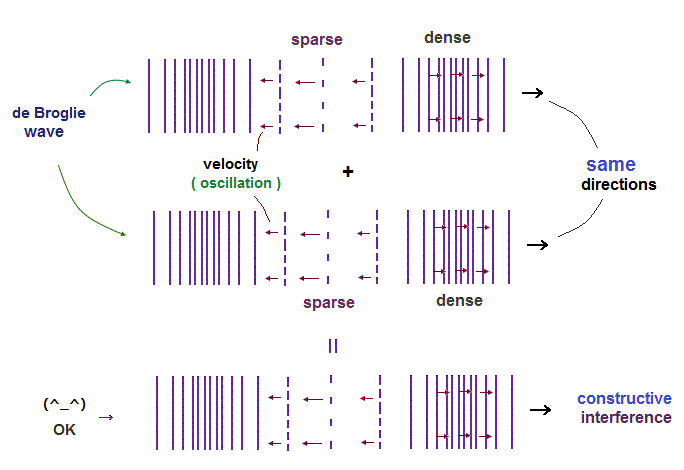When the same phases of two de Broglie waves in the same direction approach each other ( sparse-sparse, and dense-dense ), they interfere with each other constructively.

Because each oscillating direction in longitudinal wave agrees with each other.

## Same direction, "different" phases cause destructive interference.

(Fig.14)  "Sparse" and "dense" areas interfere destructively.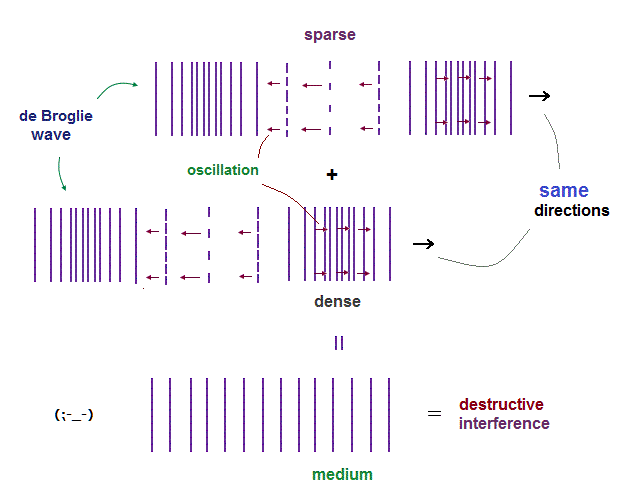But when different phases ( sparse - dense ) of two de Broglie waves overlap, they interfere destructively. In this case, each electron repels each other, as shown in double-slit interference experiment.

## de Broglie waves in the "opposite" directions "crash" and repel each other.

(Fig.15)   The opposite de Broglie waves contain the "opposite" oscillations.When two de Broglie waves in the opposite directions approach each other, they have the opposite oscillation in each phase, so crash and repel each other.

(Fig.16)   The opposite de Broglie waves = destructive interference.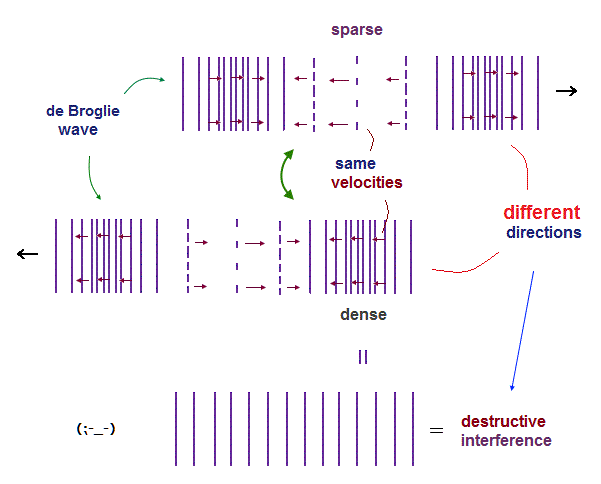As shown in Fig.16, de Broglie waves in the opposite directions contains the same oscillation in the different phases ( sparse-dense ).

So after all, the opposite de Broglie waves end in destructive interference, which gets fields back to the original "medium"-density .

## Circular orbit of one de Broglie wavelength.

(Fig.17)  One de Broglie wavelength orbit consists of sparse and dense parts.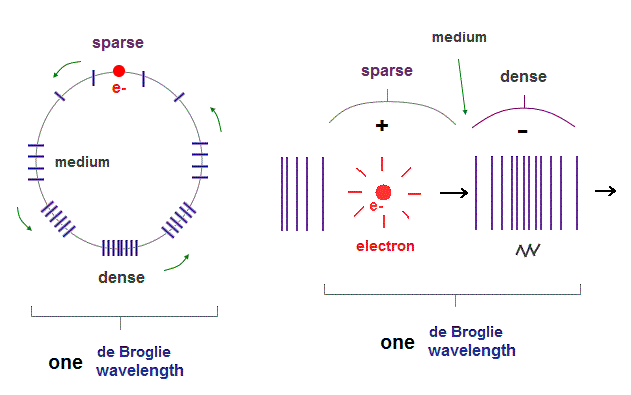Fig.17 right is an one de Broglie wavelength circular orbit, which consists of a pair of "sparse" and "dense" parts.  "Medium"-dense parts are in the middle between them.

## Interference of de Broglie waves in a circular orbit.

(Fig.18)  Interference of de Broglie waves in "different" directions.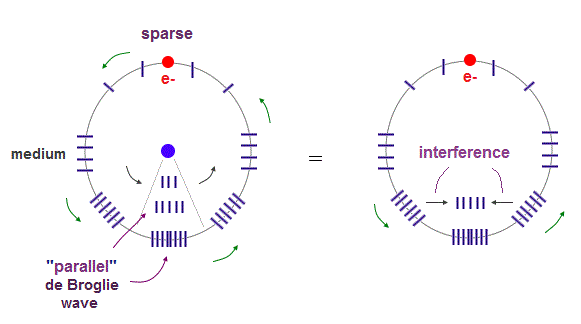Inside the circular orbit, the direction of de Broglie waves become parallel to de Broglie waves closest to them on the circumference.

As shown in Fig.18 right, we can consider two de Broglie waves in different directions generate one new de Broglie wave just between them.

## de Broglie waves in the opposite directions "cancel" each other.

(Fig.19)  Interference of two de Broglie waves generates one new de Broglie wave.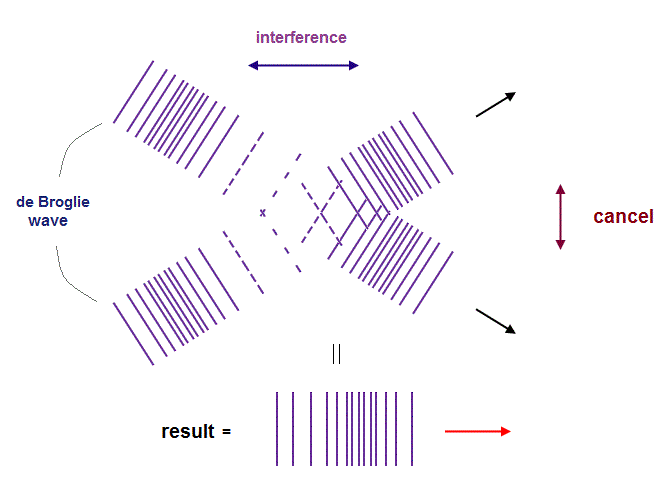As I said in Fig.15 and Fig.18, two de Broglie waves in the opposite directions cancel each other, due to their opposite oscillations.

In Fig.19, only vertical components of de Broglie waves are the opposite, so cancel each other.
As a result, new de Broglie wave in the horizontal direction is generated by this interference.

## Interference inside "elliptical" orbit.

(Fig.20)  de Broglie waves in "elliptical" orbit.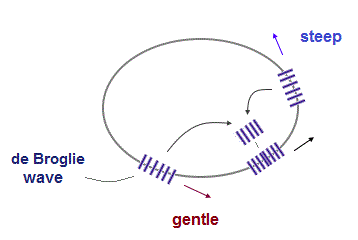In the interference inside "eplliptical" orbit, we have to consider the "angle" of each de Broglie wave.   In Fig.22, "gentle" and "steep" de Broglie waves on the circumference interfere with each other between them.

## "Moving" electric fields (= de Broglie waves ) and magnetic force.

(Fig.21)  Moving electric fields with different strength cause different magnetic force.As I said in Fig.8, we can explain this interference considering the movement of the electric field lines radiating outwards ( or inwards ) from electric charges.

In Fig.8, an electron and its electric field are moving upward.
In the left area (= "1", "gentle" in Fig.20 ), the spaces of electric field lines are narrow in the propagation direction.

So the generated de Broglie waves become stronger in the narrow electric fields than the wide electroc fields (= "2", "steep" in Fig.20 ).

But the "gentle" part is farther away than "steep" part in Fig.8, which can cancel this "gentle", "steep" difference.
As a result, de Broglie wave can be parallel to that on the circumference also inside elliptical orbits.

## Biot-Savrt law can be explained by de Broglie waves.

(Fig.22)  Biot-Savart law -- magnetic and electric fields.The relation between electric fields and de Broglie waves can naturally explain Biot-Savart law.
In Biot-Savart law, the magnetic fields become stronger at points which are perpendicular to the electric currents.

This relation between magnetic force strength and currents is just equal to that between the electric field's spaces (= narrow or wide ) and de Broglie waves.

Using this mechanism ( considering the distance, too ), we can explain the interference inside elliptical orbits.

## 2 × de Broglie wavelength orbit contains two midlines.

(Fig.23)  2 × de Broglie wavelength can contain two electrons.2 × de Broglie wavelength orbit consists of two pairs of electrons and its opposite phases. And it means two midpoint lines (= zero phase ) are included.

## Periodic motions of Neon eight valence electrons.

(Fig.24)  Four orbits cross each other "perpendicularly" in Neon.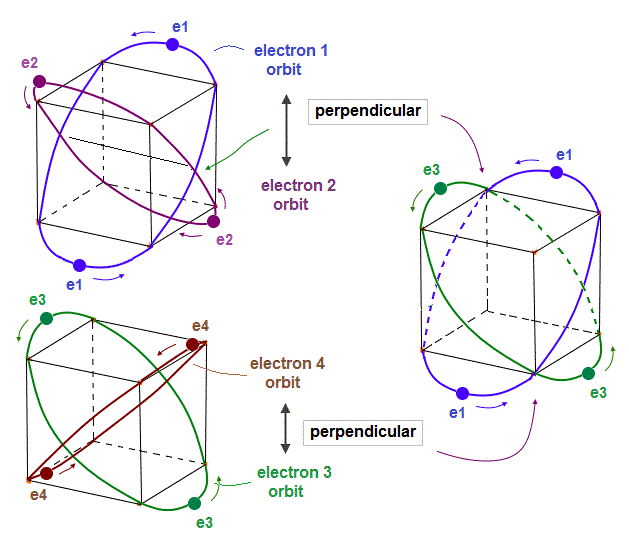On this page, we can explain Neon using four orbits crossing each other.

(Fig.25)  Periodic motions of Neon eirht valence electrons (= four orbits ).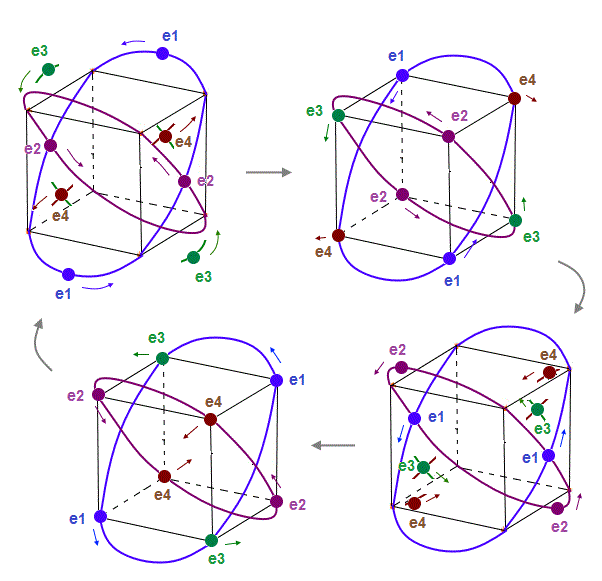As shown in Fig.25, we can show the periodic motions of Neon eirht valence electrons included in four symmetrical orbits.

## Reason why "Neon" contains "4 orbits" stably.

(Fig.26)  2 perpendicular orbits + 2 midpoint lines = 4 orbits in Neon.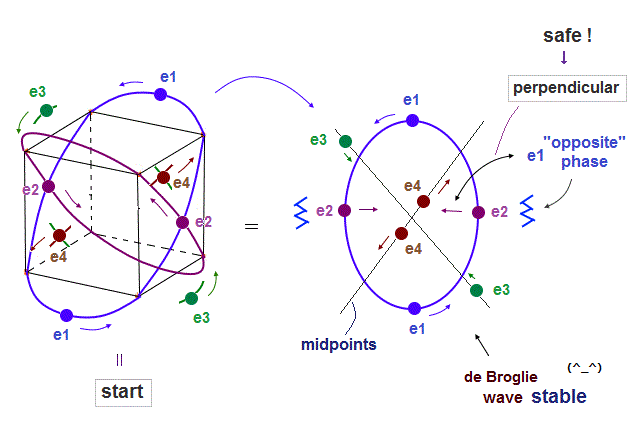We can explain new Neon model using 4 orbits, each contains two electrons ( 2 × 4 = 8 valence electrons ).  Each two orbits form a pair, when they cross perpendicularly.

When "two orbits" (= e1, e2 ) cross perpendicularly in each pair, other orbits (= e3, e4 ) are NOT perpendicular to e1, e2.

For these e3 and e4 electrons to move stably, their de Broglie waves must not be disturbed.
So e3 and e4 electrons must be on the midpoint lines of e1 and e2 to avoid destructive interference.

2 × de Broglie wavelength orbit contains two midpoint lines.
As a result, Neon can contain the maximum 4 orbits (= 2 crossing + 2 midlines ).

## "Midpoints" are necessary for preventing electron's de Broglie waves from being disturbed.

(Fig.27)  When "e3" is just between "e1" and "e2", it must pass "midpoints".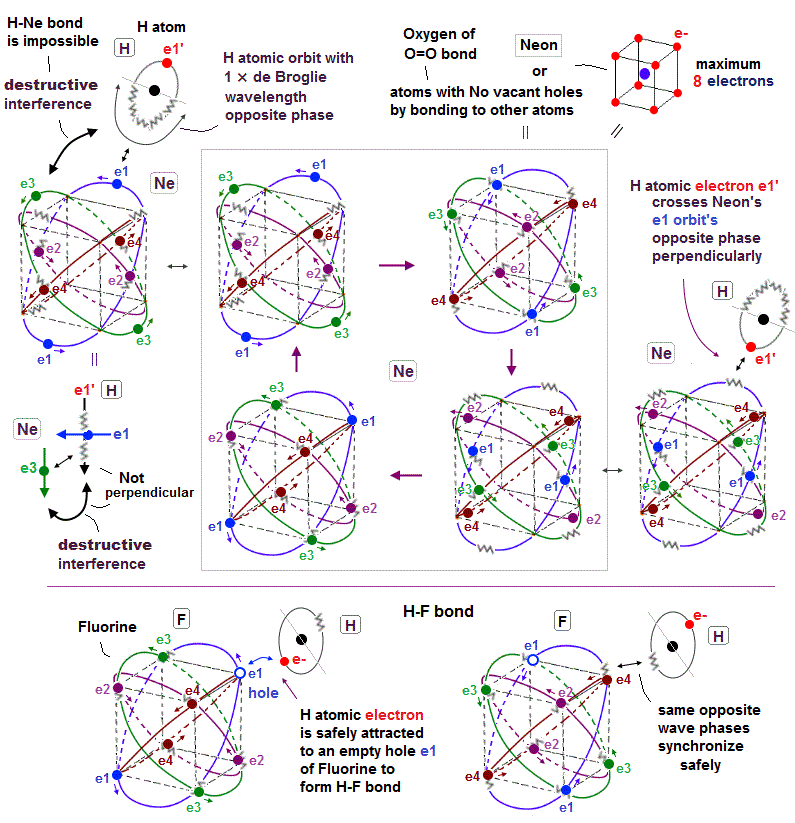In Fig.27 middle, "e3" electron just passes the point wich is the same distance from e1 and e2 orbits.
At this point, e3 de Broglie wave can be perpendicular to neither of "e1" and "e2" orbits.

To prevent disturbing "e3" de Broglie waves, two symmetrical de Broglie waves of e1 and e2 must be cancelled out.  As shown in Fig.26, at this time, "e3" is just on the midpoint lines in both of e1 and e2 orbits.

As shown in Fig.22 (= de Broglie waves and Biot-Savart law ), when an electron crosses another orbit, this electron is influenced only by this orbit (= "perpendicular" to each otther ), because "crossing" means their distance is zero, which makes its de Broglie wave power the strongest.

## Number of "midpoints" lines determines the total number of orbits.

(Fig.28)  When "e3" is just between e1 and e2, it must pass midpoints.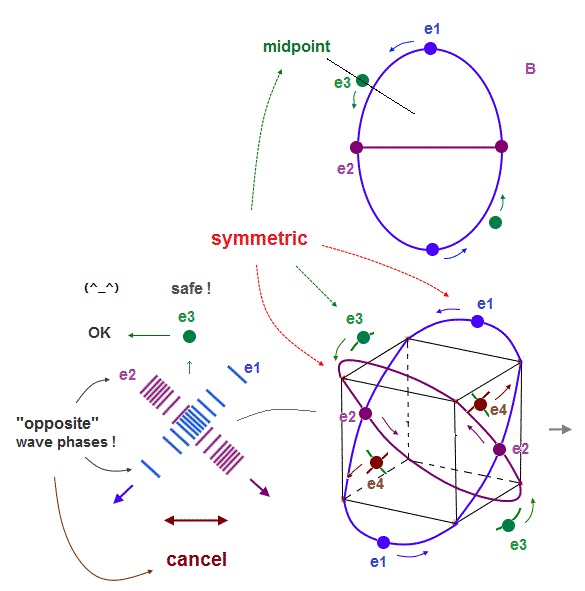As shown in Fig.28, "e1" and "e2" orbits are just symmetrical with respect to "e3", when it pass the point which is the same distance from e1 and e2.

As I said in Fig.27, the wave phases from "e1" and "e2" must be cancelled out to be zero at this point NOT to disturb "e3" de Broglie waves.  So the wave phases of e1 and e2 must be just the opposite (= ± ), or both zero.

The important point is that e1 and e2 orbits must be symmetrical ( considering Coulomb force ) with respect to "e3". So there is the only one choice in which both e1 and e2 are midpoints at this point.

If "e1" wave phase is "+" and "e2" wave phase is "-", it is NOT symmetrical from the viewpoint of "e3", which causes different shaped "e3" orbit.

## Two 1 × de Broglie wavelength orbits cross "perpendicularly", avoiding "destructive" interference.

(Fig.29)  "Opposite" wave phases interfere with each other, destructively. → "perpendicular".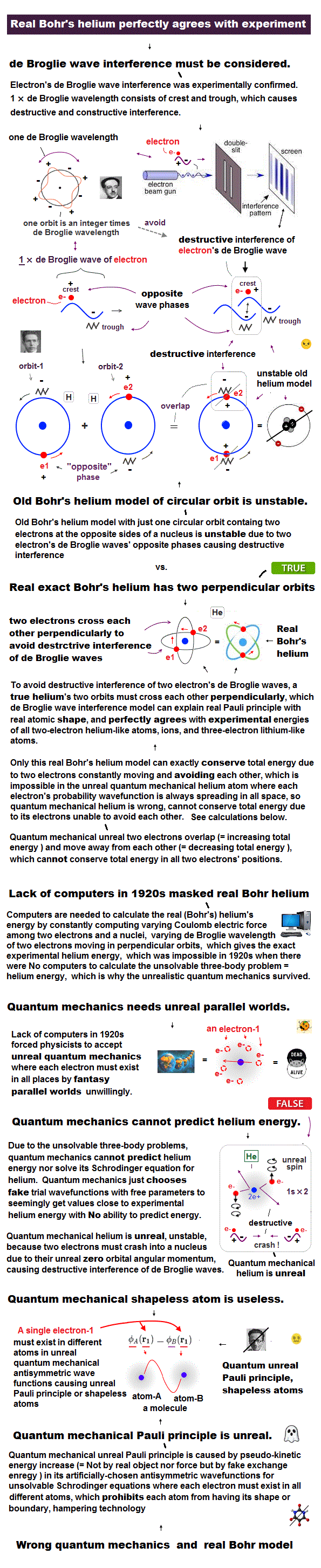Helium atoms contains two 1 × de Broglie wavelength orbits ( n = 1 ).
So, two opposite wave phases cause "destructive" interference and instability.

To avoid this destructive interference, two orbits of helium have to cross each other "perpendicularly", because "perpendicular" means each wave phase can be independent from another.

## "Perpendicular" crossing of helium orbits.

(Fig.30)  Two electrons have to pass each other on "midpoint" lines.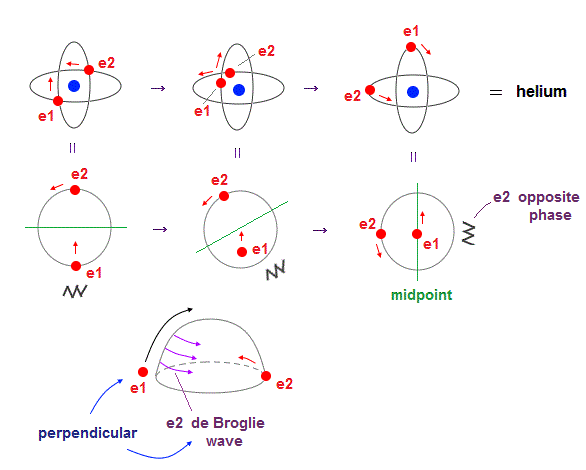In one de Broglie wavelength orbit, a half of it is "opposite" wave phase.
So, "e1" electron of Fig.4 must go in the direction perpendicular to "e2" orbit to midpoint.

Two electrons in "perpendicular" orbits have to pass each other in the "parallel" direction at "midpoint", because "midpoint" line is zero phase, which has NO influence on another wave.

## "Midpoint" line is "medium" phase, which has NO influence on other waves.

(Fig.31)  Midpoint between ±opposite phases has NO influence on another wave.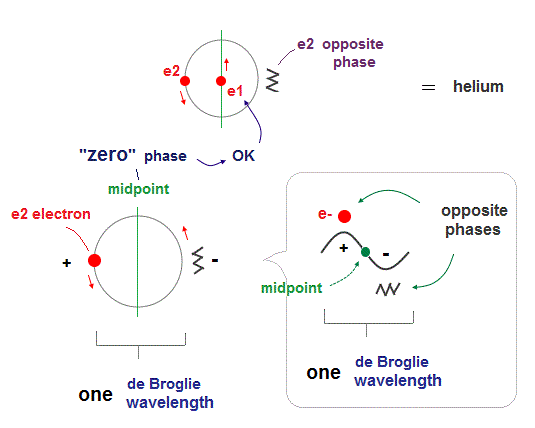"Midpoint" line between ±opposite phases contains "medium (= neutralized )" zero phase.
So this midpoint has NO influence on another wave phase (= NOT disturb other de Broglie waves ).

So, de Broglie wave can pass each other in the parallel direction, NOT disturbed on the midpoint lines.2015/4/19 updated. Feel free to link to this site.